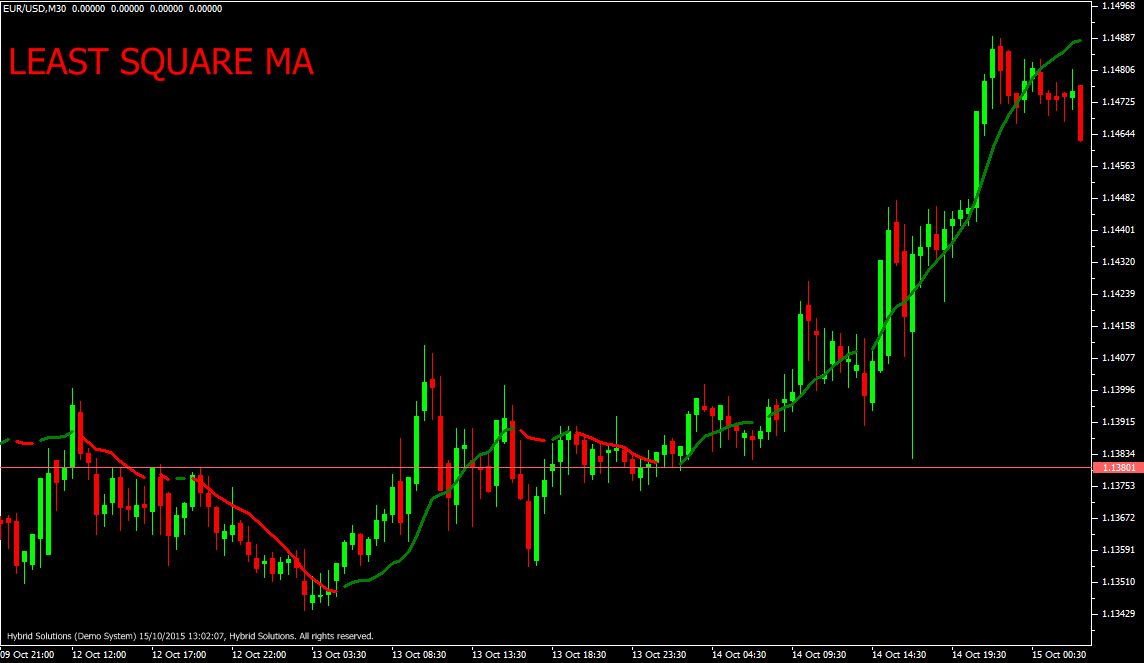# Least Square MA

Free

Developed By: NeeduVerdeSolution

In stock

Least Square MA is a moving average based VertexFX client side VTL indicator that employs least-square’s, best-fit model. In the least squares best-fit methodology, given a number of points, we find an ideal line which is close to all points.

SKU: 773 Categories: ,Least Square MA is a moving average based VertexFX client side VTL indicator that employs least-square’s, best-fit model. In the least squares best-fit methodology, given a number of points, we find an ideal line which is close to all points.

The same concept is extended to this indicator. Given the most recent R_PERIOD closing price points, we find an ideal line which is close to all the R_PERIOD points. The Y axis value of this ideal line is calculated for the current point, and this is the indicator value. Using the closing prices, we calculate the least squares co-efficient (SLOPE and INTERCEPT) over the recent R_PERIOD candles. This co-efficient is calculated by using the Linear Weighted Closing price of the recent R_PERIOD bars. In the next step, we calculate the projected value on the ideal line on the current bar. This process is repeated for each bar to generate the Least Square MA. The indicator is GREEN when it is rising and RED when it is falling. The Least Square MA builds the price curve which is close to all the candle closes over the R_PERIOD. Hence it reduces the lag efficiently.

The idea behind the indicator is that in trending market, the indicator will fit smoothly (because recent prices are either moving upwards or downwards), and hence the difference between the current price and the indicator value will be small. In sideways markets, this difference will be large, and leaves room for whip-saws and safe stop losses.

BUY – Place Buy position when indicator value turns GREEN. Avoid entering trade when candle gaps up.
SELL – PlaceSell when indicator value turns RED. Avoid entering trade when candle gaps down.